# mathr.co.ukcalendar2018

Now available for purchase at lulu.com!

## Cover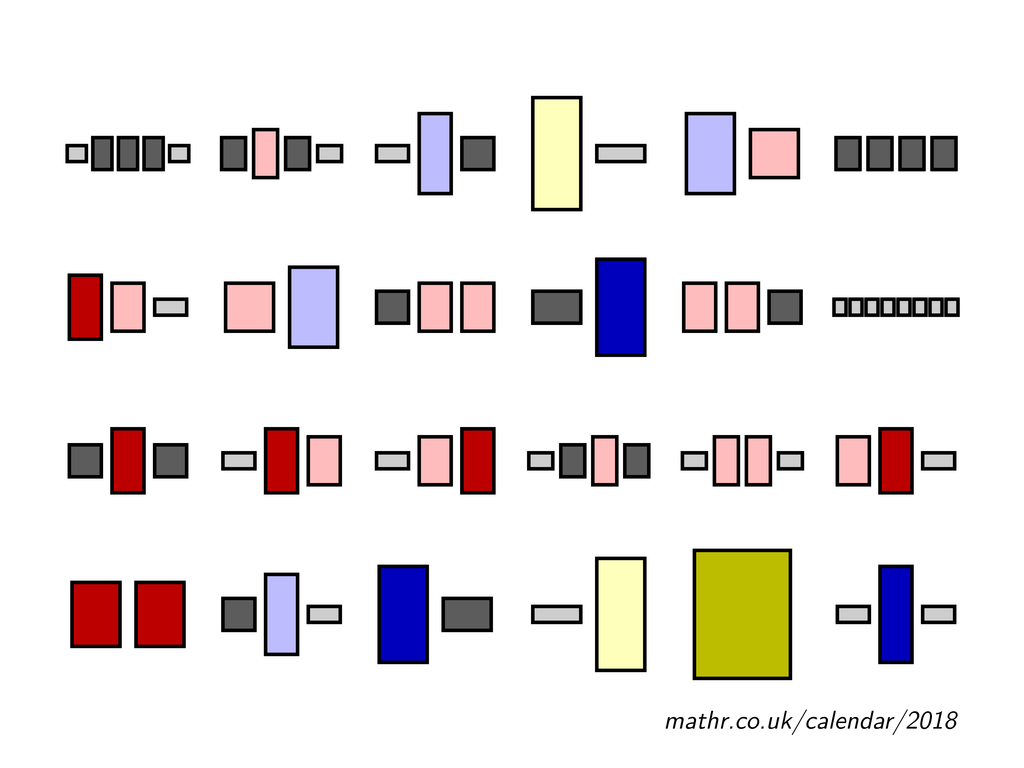### A070211

a(n) is the number of compositions (ordered partitions) of n that are concave sequences.

a(8) = 24 is illustrated; a(35) = 2018

## January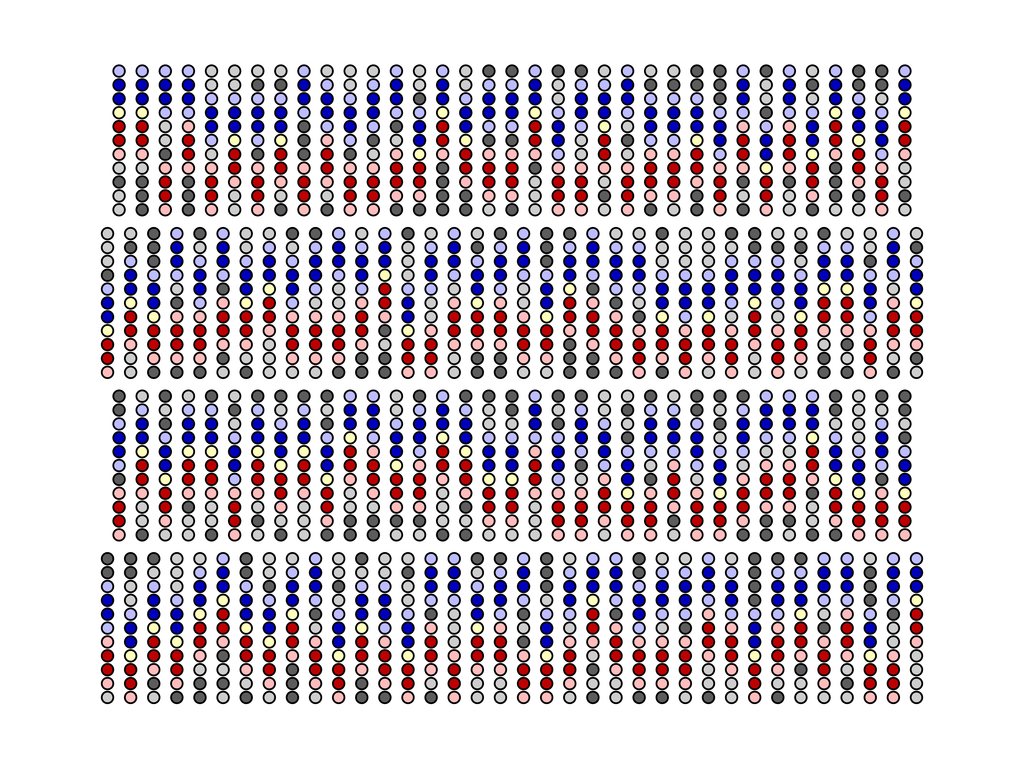### A118890

T(n,k) is the number of binary sequences of length n containing k subsequences 0110.

a(25) = T(11,2) = 142 is illustrated; a(38) = T(14,2) = 2018

## February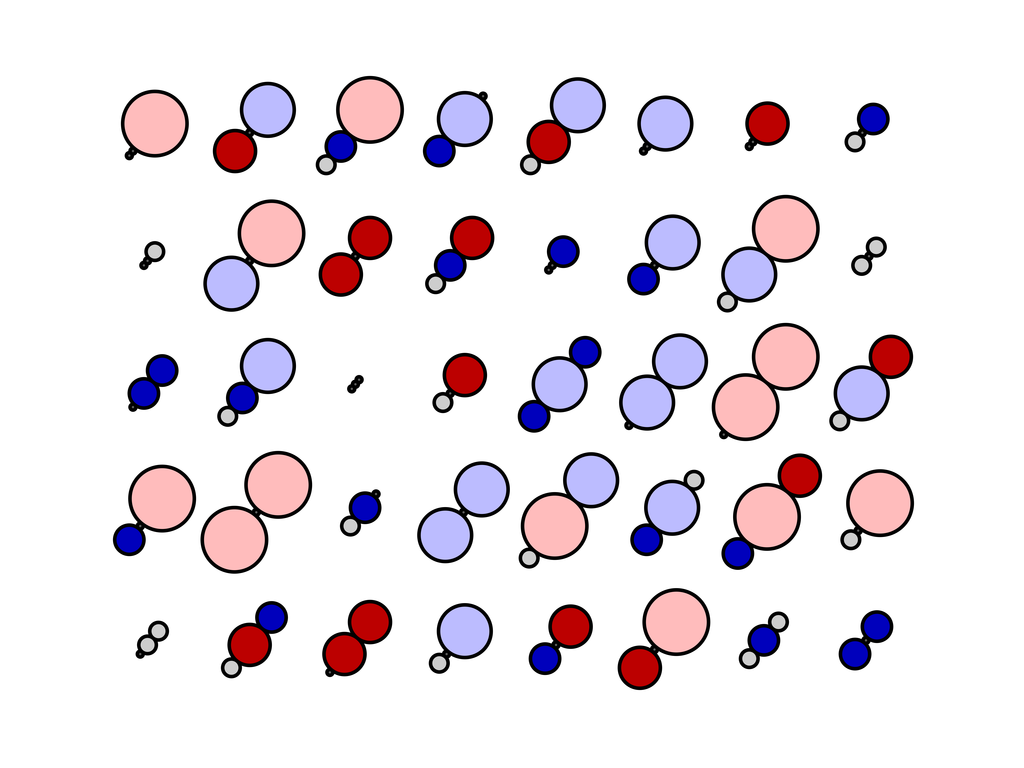### A213497

a(n) is the number of (w,x,y) with all terms in {0,...,n} and w = min(|w-x|,|x-y|).

a(5) = 40 is illustrated; a(40) = 2018

## March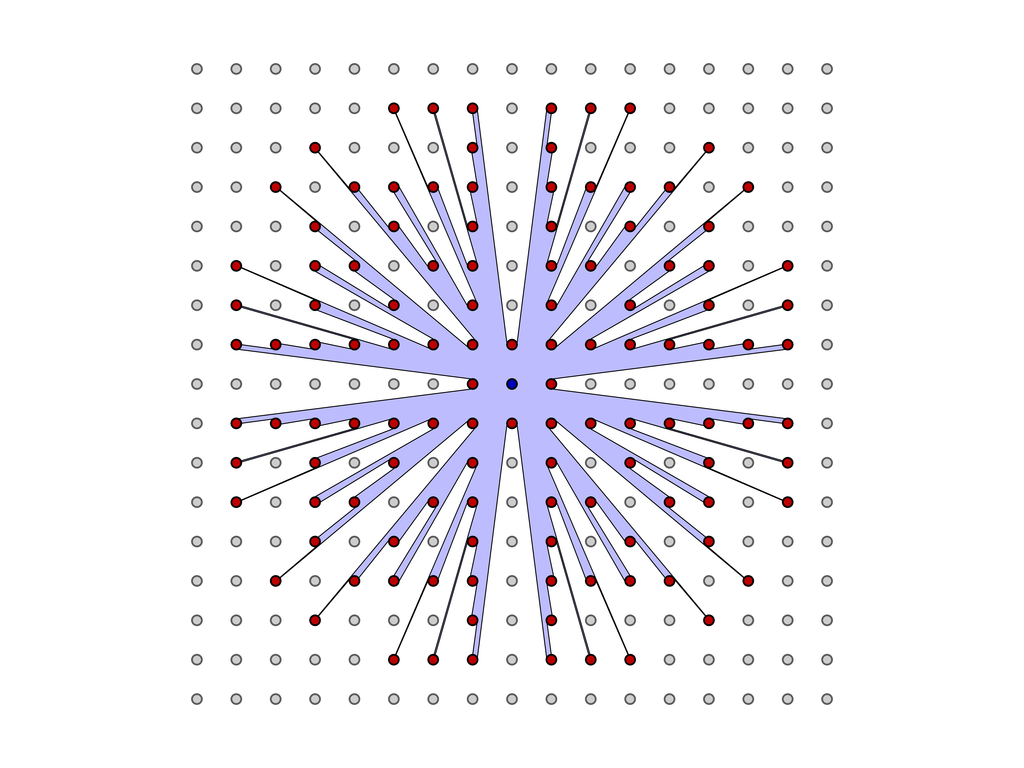### A124255

a(n) is the square of the distance to most distant visible tree of radius 1/n.

a(8) = 61 is illustrated; a(45) = 2018

## April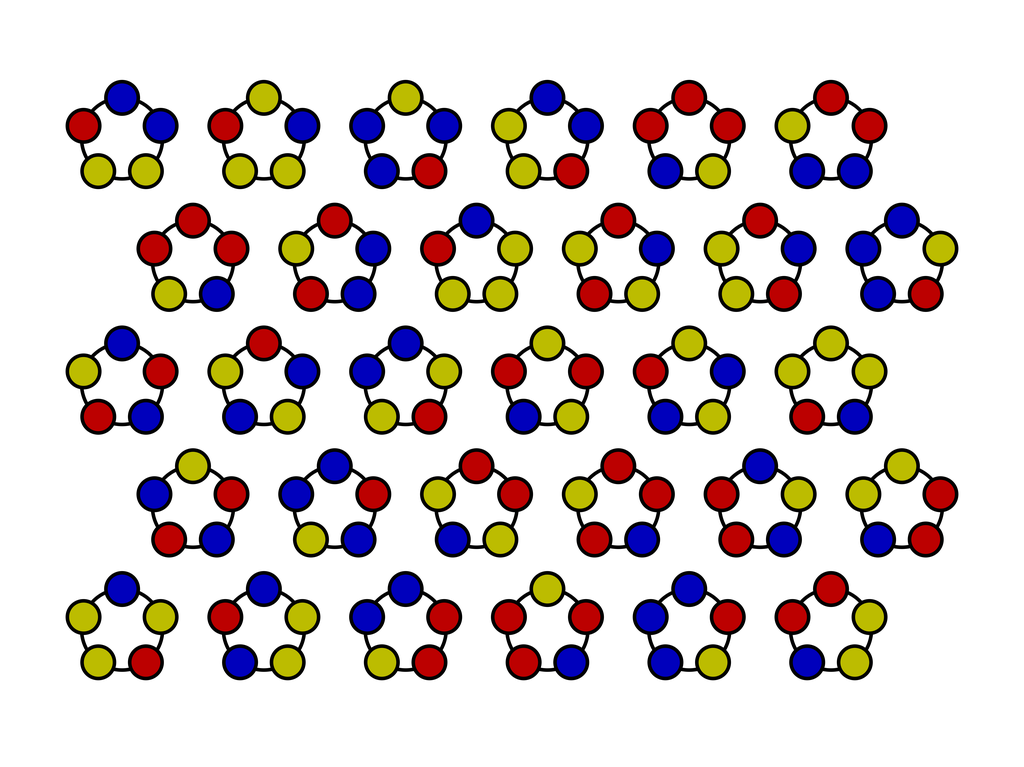### A056283

a(n) is the number of n-bead necklaces with exactly three different colored beads.

a(5) = 30 is illustrated; a(8) = 2018

## May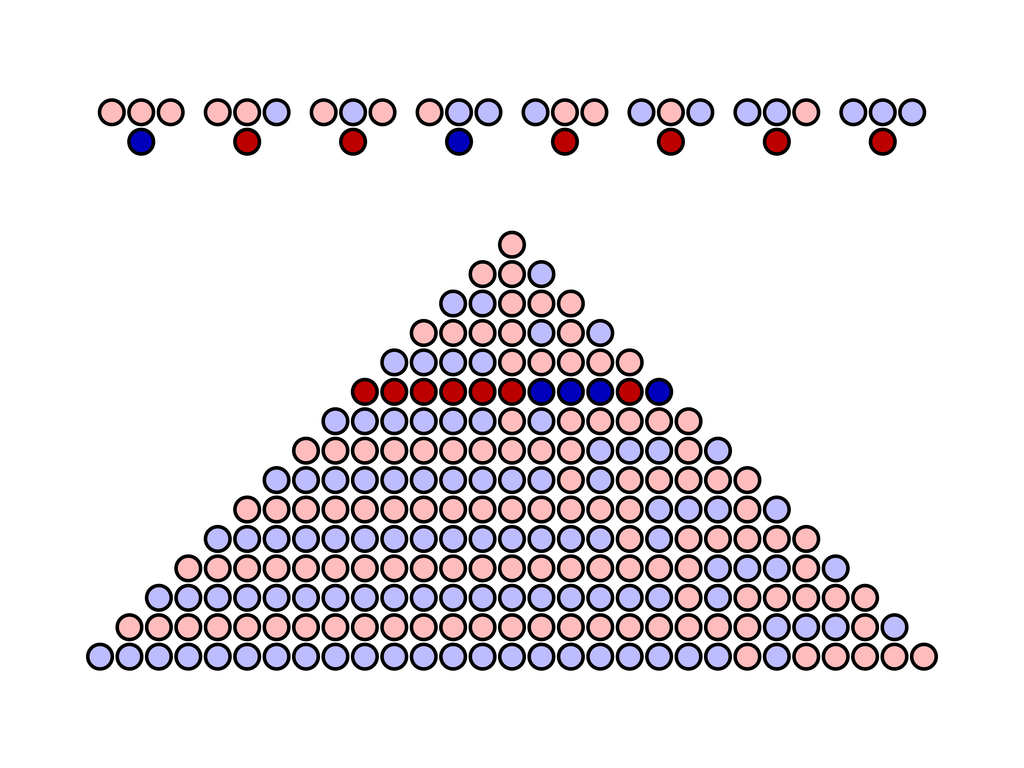### A267255

a(n) is the decimal representation of the n-th iteration of the "Rule 111" elementary cellular automaton.

a(5) = 2018 is illustrated

## June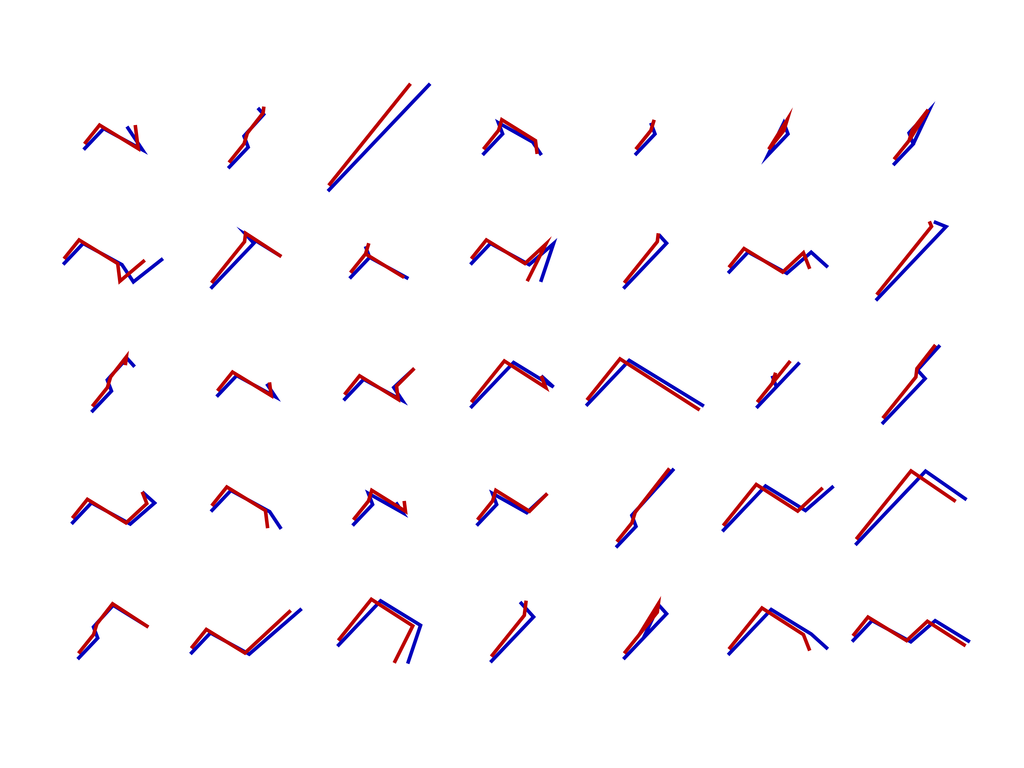### A149037

a(n) is the number of walks within N^3 (the first octant of Z^3) starting at (0,0,0) and consisting of n steps taken from
{(-1, -1, 0), (-1, 0, 1), (0, 1, 1), (1, -1, 1), (1, 0, -1)}.

a(4) = 35 is illustrated; a(7) = 2018

## July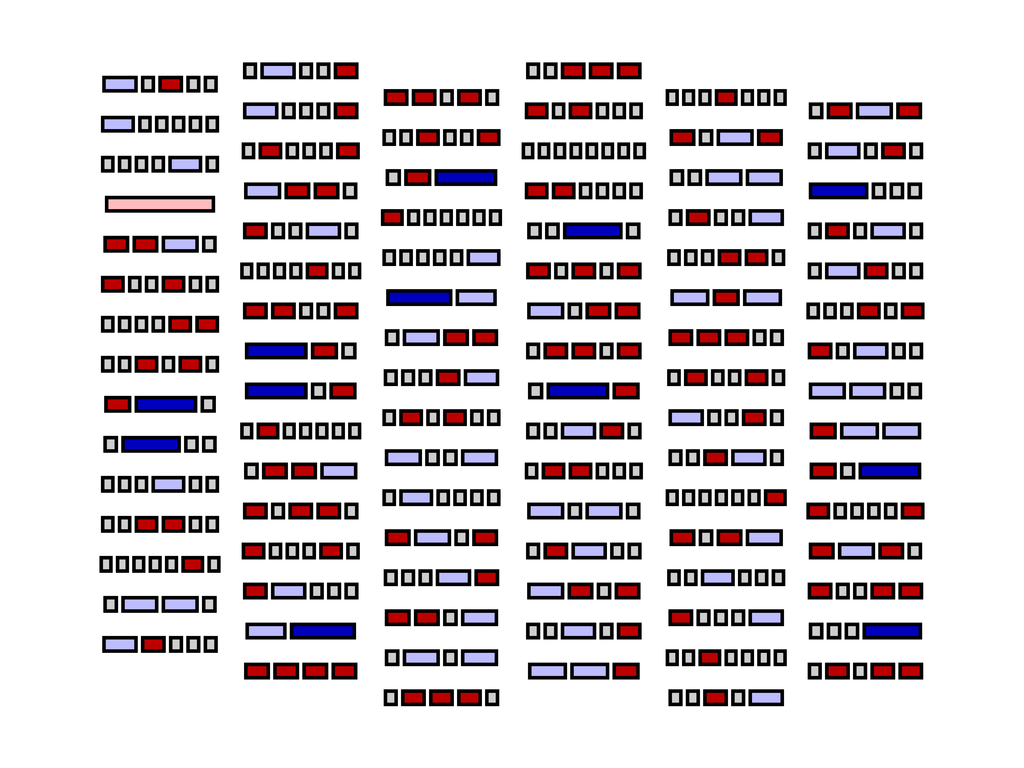### A121551

a(n) is the number of parts in all the compositions of n into Fibonacci numbers.

a(8) = 457 is illustrated; a(10) = 2018

## August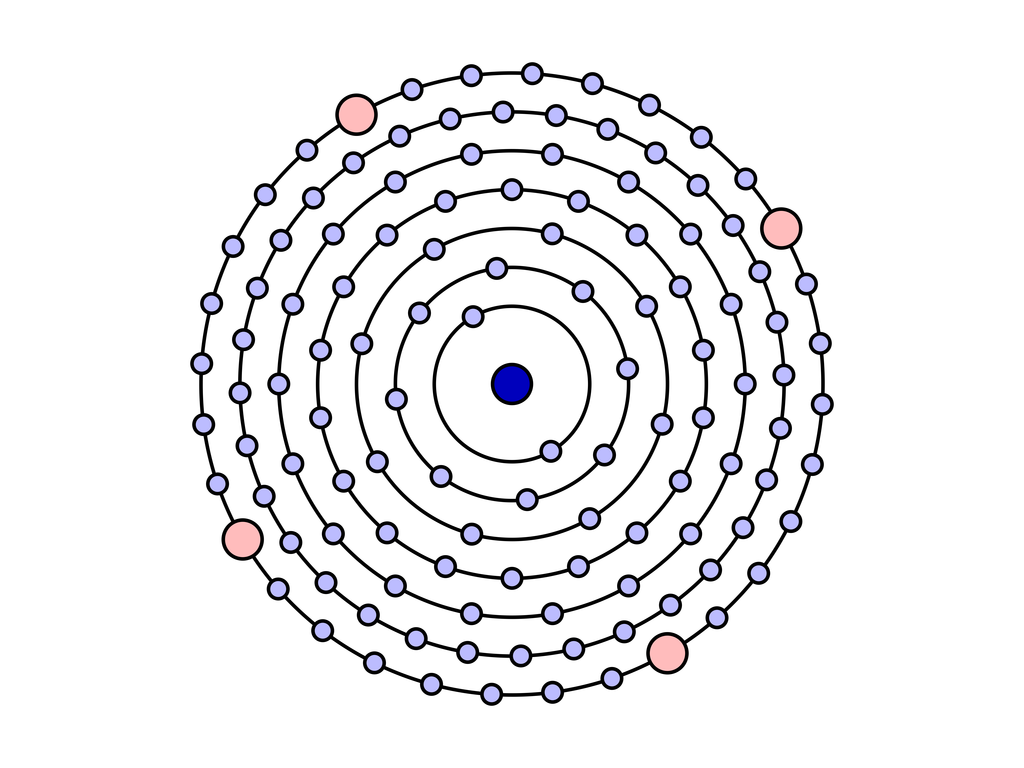### A271996

a(n) is the crystallogen sequence.

a(7) = 114 is illustrated; a(21) = 2018

## September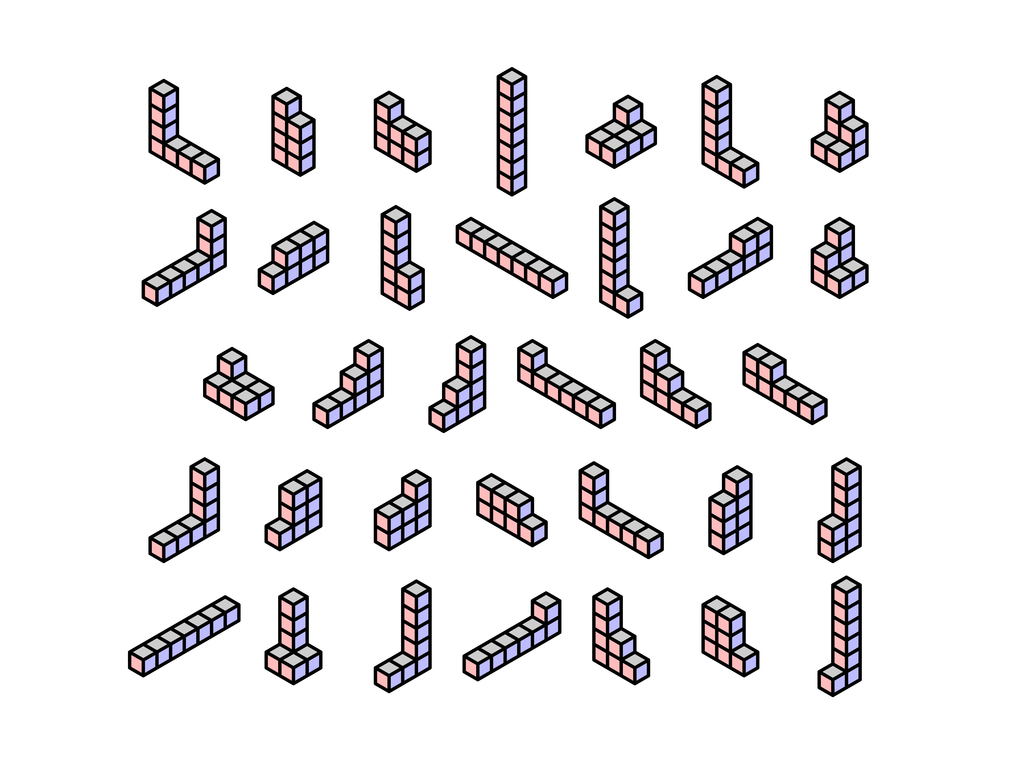### A229915

a(n) is the number of espalier polycubes of a given volume in dimension 3.

a(7) = 34 is illustrated; a(20) = 2018

## October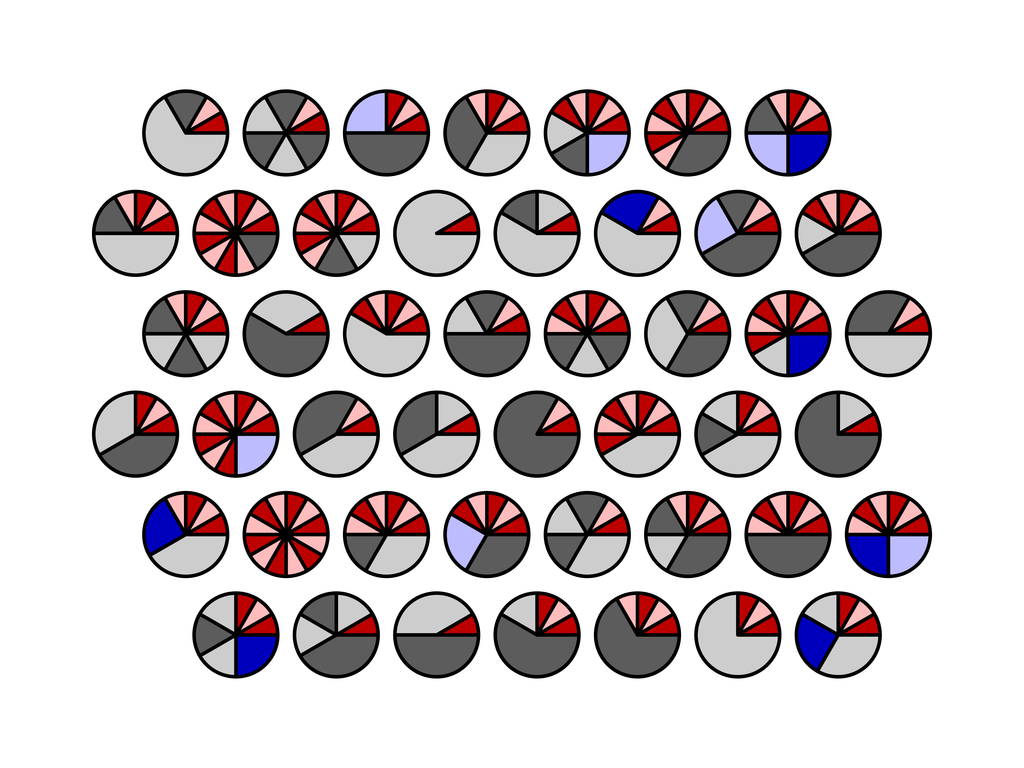### A240059

a(n) is the number of partitions of n such that multiplicity(1) > multiplicity(3).

a(12) = 46 is illustrated; a(27) = 2018

## November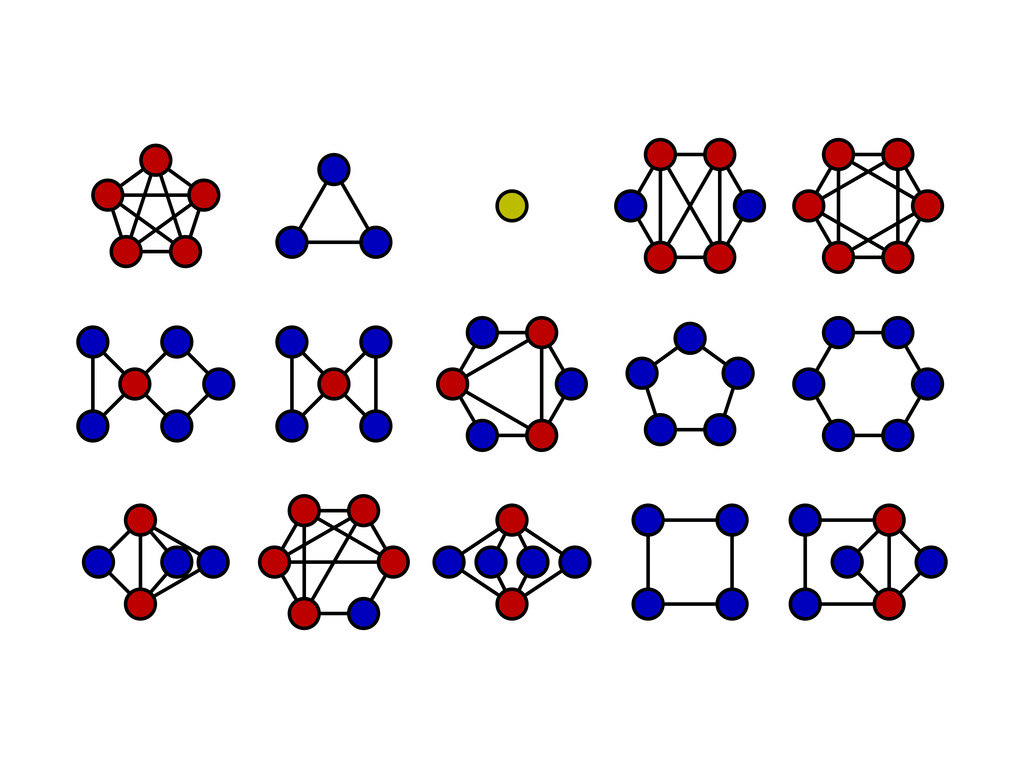### A133736

a(n) is the number of graphs on n unlabeled nodes that have an Eulerian cycle,
i.e., a cycle that goes through every edge in the graph exactly once.

a(6) = 15 is illustrated; a(9) = 2018

## December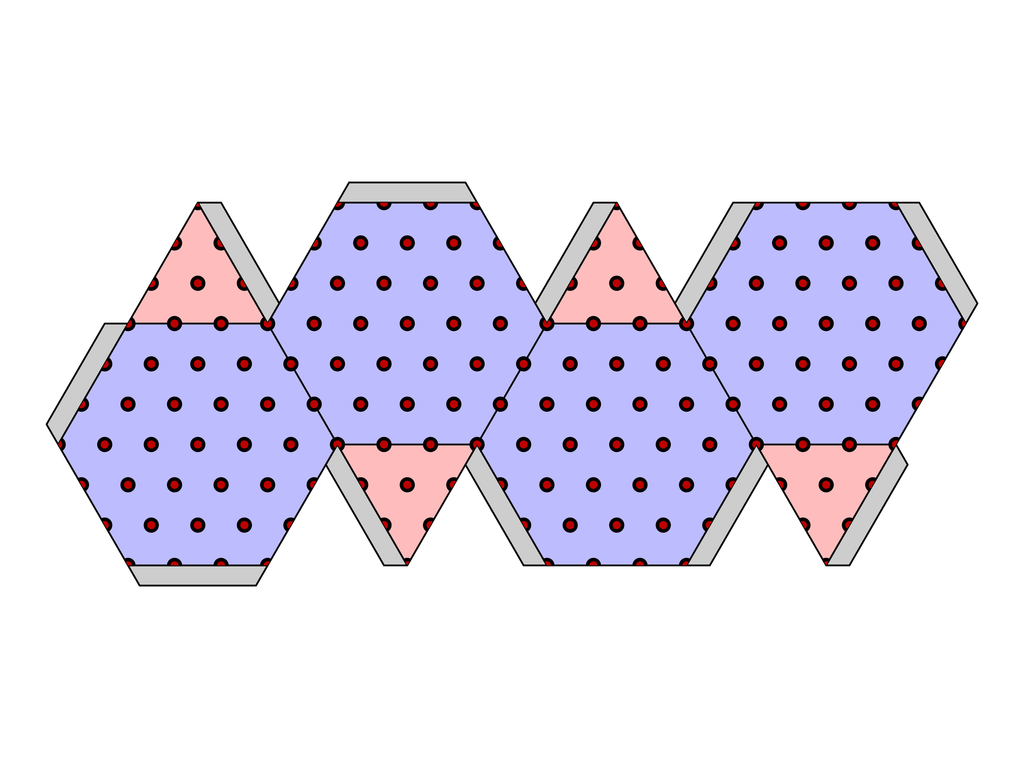### A005905

a(n) is the number of points on the surface of a truncated tetrahedron of edge length n (by analogy with triangular numbers).

a(4) = 226 is illustrated; a(12) = 2018

Each of the 13 designs (12 months plus cover) is based on a sequence from the Online Encyclopedia of Integer Sequences® in which the number 2018 occurs. OEIS is a registered trademark of the OEIS Foundation, Inc. This project is neither endorsed by nor affiliated with the OEIS.

## source code

source code is available, implemented in Haskell using the Diagrams library:
`git clone https://code.mathr.co.uk/oeis-diagrams.git`

back to top

mathr.co.uk